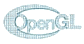# March - A Marching Cubes Implementation## The Idea

Visualisation of volume data can be done in many ways. One of this ways is the Marching Cubes Algorithm. The program presented here implements this algorithem. This work is done for the Lab "Visualisierung" at the Institute of Computer Graphics and Algorithms.

## The Algorithm

The algorithm describes the visualisation of medical volume data. It processes multible 2D slices given by procedures like the Computer Tomography (CT) or the Magnetic Resonance (MR). The result of such procedures are so called Voxels.

The first step is normalizeing the volume data. Then the algorithm takes eight adjacent voxels, i.e. four voxel from each of two adjacet slice, and puts dem on the corners of a cube. Then it determines which of these corners is outside or inside the the volume that should be visualised (given by a threshold). So each corner gets a one, if it is inside or on the sucface and zero otherwise. Eight corners, two values each leads to 256 types of cube configurations. Considering complementary cases and symmetries reduces these 256 types to 14 basic types.

The basic types now describe, which surface elements the corner configuration produces. Entering the corners value in a eight bit integer helps to decide quickly of which type the cube is, and the basic types give the surface triangles the cube is producing. The triangles vertices are interpolated along the edges of the cube. The last step is the calculation of the sucface normals for each vertex of each triangle. This is done by the following equations:

``` Gx(i,j,k) = (D(i+1,j,k) - D(i-1,j,k))/dx Gy(i,j,k) = (D(i,j+1,k) - D(i,j-1,k))/dy Gz(i,j,k) = (D(i,j,k+1) - D(i,j,k-1))/dz ```

Where `D(i,j,k)` is the density of the voxel at position `(i,j)` in slice `k` and `dx,dy,dz` are the lengths of the cube edges.

## The Program

The program is completely written in C++. I used the Cygwin environment to create a Windows executable. All graphics code is done in OpenGL.

### Basic Functionallity

The program reads voxel data and applies the Marching Cubes algorithm on it. It creates a GL triange mesh and displays it. The displayed object can be turned along all axis and zoomed.

### Extended Functionallity

Several extentions are possible:

• voxel reduction by a multiple of two,
• introduction of clippint planes into the algorithm,
• displaying two or more survaces with the outer surface displayed semitransparent,
• interactive change of thresholds.

The last extention could lead to problems, because the algorithm prepares the survace. A change of threshold causes the algorithm to scan the whole data and produce a new triangle mesh, so maby this is'nt very interactive.

## The Code

The code is written in several steps. The first step is the implementation of an stable Marching Cubes algorithm. The algorithm creates a point list and a triangle list consisting of point list indices. The next step is displaying this data. And the last step is to extend the functionallity of the above mentioned program parts.